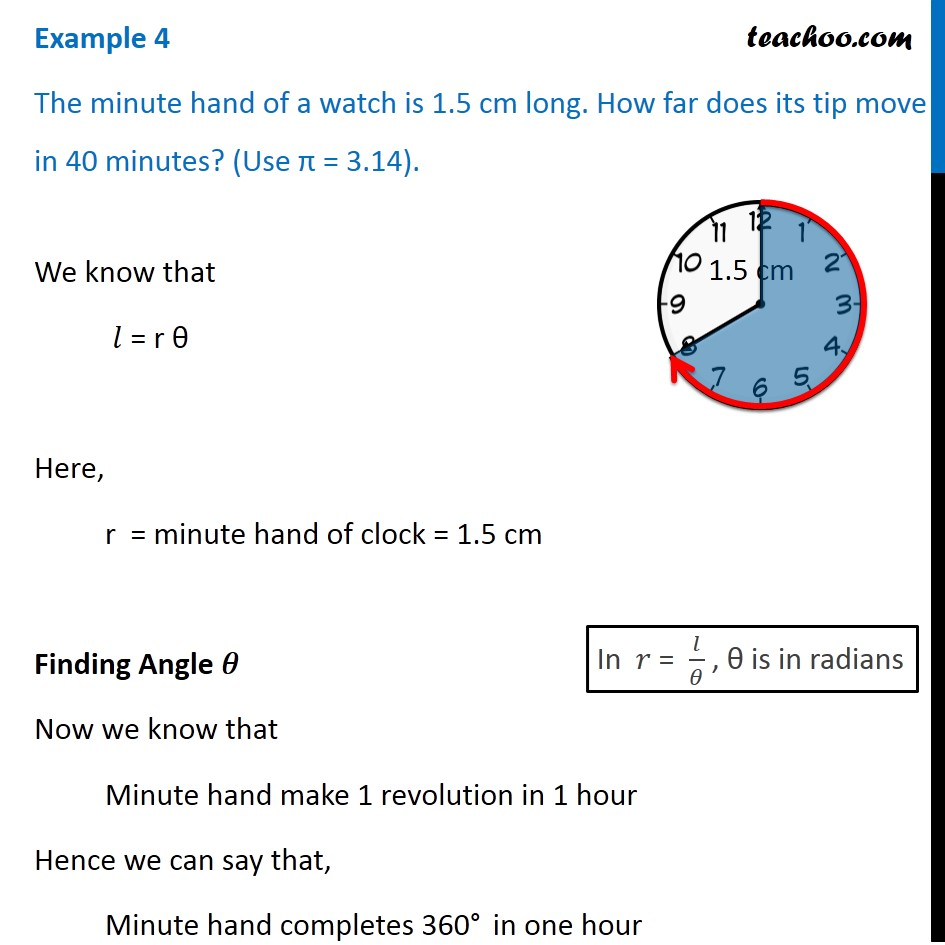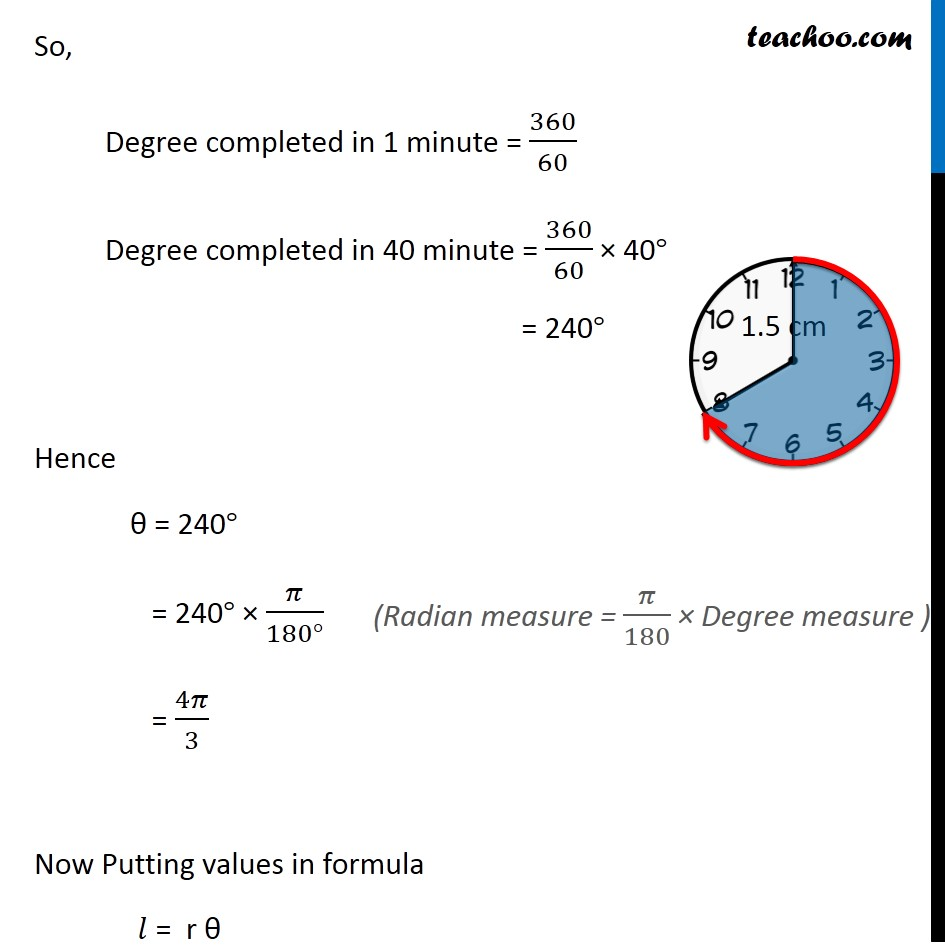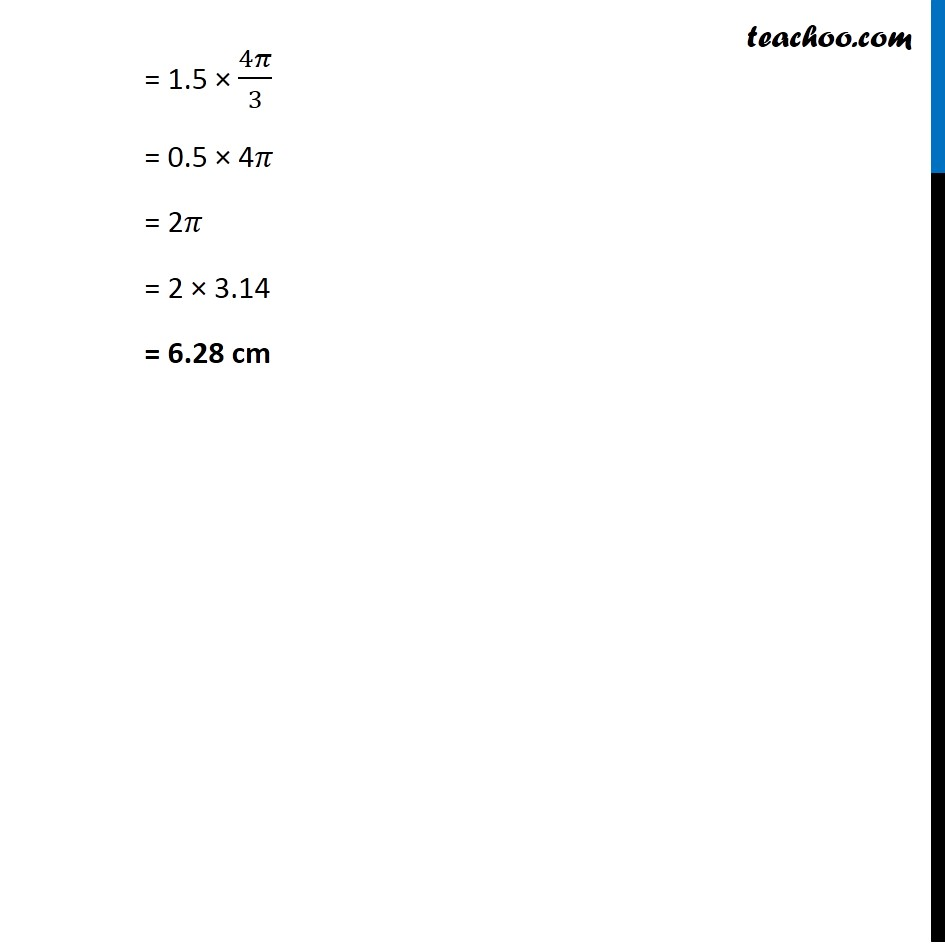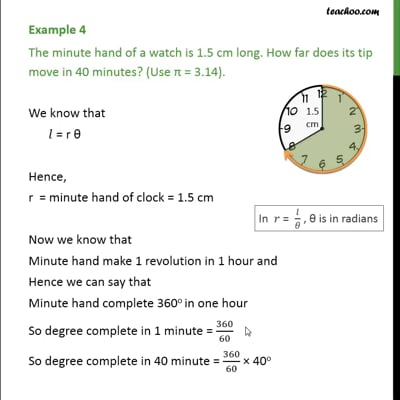Examples

Chapter 3 Class 11 Trigonometric Functions (Term 2)
Serial order wiseThis video is only available for Teachoo black users

### Transcript

Example 4 The minute hand of a watch is 1.5 cm long. How far does its tip move in 40 minutes? (Use π = 3.14). We know that 𝑙 = r θ Here, r = minute hand of clock = 1.5 cm Finding Angle 𝜃 Now we know that Minute hand make 1 revolution in 1 hour Hence we can say that, Minute hand completes 360° in one hour In 𝑟 = 𝑙/𝜃 , θ is in radians So, Degree completed in 1 minute = 360/60 Degree completed in 40 minute = 360/60 × 40° = 240° Hence θ = 240° = 240° × 𝜋/(180°) = 4𝜋/3 Now Putting values in formula 𝑙 = r θ (Radian measure = 𝜋/180 × Degree measure ) = 1.5 × 4𝜋/3 = 0.5 × 4𝜋 = 2𝜋 = 2 × 3.14 = 6.28 cm